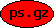Completion to Involution and Semi-Discretisations Reference: Applied Numerical Mathematics, 42 (2002) 437-451 Description: This paper studies what is happening, if one semi-discretises a linear over-determined system of partial differential equations. I show that for a certain class of systems, the arising differential algebraic equation will be formally integrable (of index 0), if and only if the partial differential system is involutive. Formal integrability of the partial differential system is not sufficient. I also analyse some aspects of integrating an underlying equation; for strongly hyperbolic systems I derive estimates for the drift off the constraints. For general linear systems I show how the index of a differential algebraic equation obtained by semi-discretisation can be predicted by completing a perturbed form of the linear system to involution. This relates index definitions in the literature (which are usually based on such semi-discretisations) with the intrinsic definition based on the formal theory I have given in the article  . Compressed Postscript File:(61 kB) Home, Last update: Thu May 27 09:48:24 2021